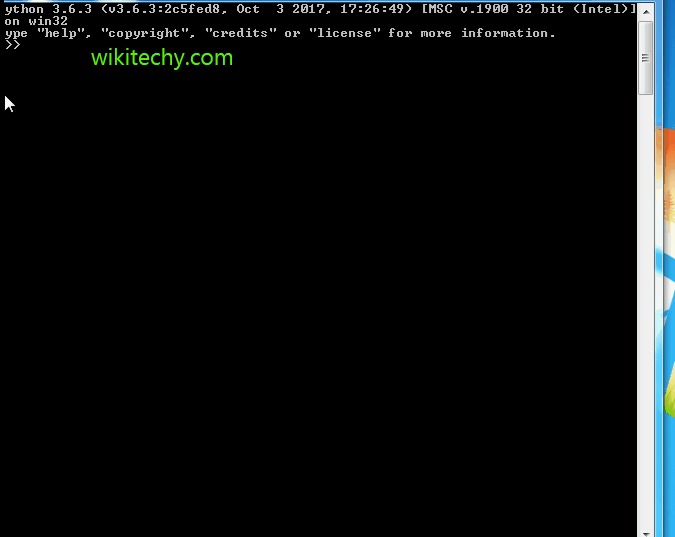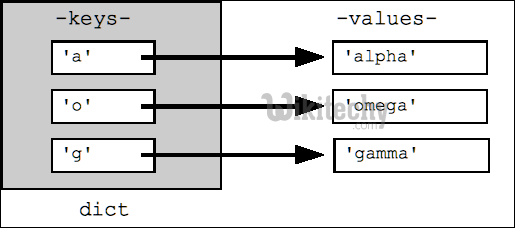# python tutorial - Python OrderedDict | OrderedDict in Python - learn python - python programmingLearn Python - Python tutorial - python dictionary orderdict - Python examples - Python programs

An OrderedDict is a dictionary subclass that remembers the order that keys were first inserted. The only difference between dict() and OrderedDict() is that:OrderedDict preserves the order in which the keys are inserted. A regular dict doesn’t track the insertion order, and iterating it gives the values in an arbitrary order. By contrast, the order the items are inserted is remembered by OrderedDict.

python - Sample - python code :

python tutorial - Output :

```This is a Dict:
('a', 1)
('c', 3)
('b', 2)
('d', 4)

This is an Ordered Dict:
('a', 1)
('b', 2)
('c', 3)
('d', 4)
```

Important Points:

1. Key value Change: If the value of a certain key is changed, the position of the key remains unchanged in OrderedDict.

python - Sample - python code :

python tutorial - Output :

```Before:

('a', 1)
('b', 2)
('c', 3)
('d', 4)

After:

('a', 1)
('b', 2)
('c', 5)
('d', 4)```

1. Deletion and Re-Inserting: Deleting and re-inserting the same key will push it to the back as OrderedDict however maintains the order of insertion.

python - Sample - python code :

python tutorial - Output :

```Before deleting:

('a', 1)
('b', 2)
('c', 3)
('d', 4)

After deleting:

('a', 1)
('b', 2)
('d', 4)

After re-inserting:

('a', 1)
('b', 2)
('d', 4)
('c', 3)

```

Wikitechy tutorial site provides you all the learn python , learn python programming online , best python course online , best online course for python , python online learning